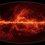# Need some help with Matrix!

For any arbitrary matrix,

$\left[ \begin{matrix} { a }_{ n+1 } \\ { b }_{ n+1 } \end{matrix} \right] \quad =\quad \begin{bmatrix} \sqrt { 3 } +1 & \quad 1-\sqrt { 3 } \\ \sqrt { 3 } -1 & 1+\sqrt { 3 } \end{bmatrix}\quad \left[ \begin{matrix} { a }_{ n } \\ { b }_{ n } \end{matrix} \right]$

where $n\in N$ Also, ${ a }_{ n }={ b }_{ n }=1$

Find ${ a }_{ 22 }$

P.S. Thanks to my friend Shantam, for the question!!Note by Abhineet Nayyar
6 years ago

This discussion board is a place to discuss our Daily Challenges and the math and science related to those challenges. Explanations are more than just a solution — they should explain the steps and thinking strategies that you used to obtain the solution. Comments should further the discussion of math and science.

When posting on Brilliant:

• Use the emojis to react to an explanation, whether you're congratulating a job well done , or just really confused .
• Ask specific questions about the challenge or the steps in somebody's explanation. Well-posed questions can add a lot to the discussion, but posting "I don't understand!" doesn't help anyone.
• Try to contribute something new to the discussion, whether it is an extension, generalization or other idea related to the challenge.
• Stay on topic — we're all here to learn more about math and science, not to hear about your favorite get-rich-quick scheme or current world events.

MarkdownAppears as
*italics* or _italics_ italics
**bold** or __bold__ bold
- bulleted- list
• bulleted
• list
1. numbered2. list
1. numbered
2. list
Note: you must add a full line of space before and after lists for them to show up correctly
paragraph 1paragraph 2

paragraph 1

paragraph 2

[example link](https://brilliant.org)example link
> This is a quote
This is a quote
    # I indented these lines
# 4 spaces, and now they show
# up as a code block.

print "hello world"
# I indented these lines
# 4 spaces, and now they show
# up as a code block.

print "hello world"
MathAppears as
Remember to wrap math in $$ ... $$ or $ ... $ to ensure proper formatting.
2 \times 3 $2 \times 3$
2^{34} $2^{34}$
a_{i-1} $a_{i-1}$
\frac{2}{3} $\frac{2}{3}$
\sqrt{2} $\sqrt{2}$
\sum_{i=1}^3 $\sum_{i=1}^3$
\sin \theta $\sin \theta$
\boxed{123} $\boxed{123}$

## Comments

Sort by:

Top Newest

Is the answer$(2\sqrt{2})^{21}$ ?

- 6 years ago

Log in to reply

Yes I also getting same ..... what's ur method ?

I did in this way......

$\\ \begin{cases} \vec { { V }_{ n } } ={ a }_{ n }i+{ b }_{ n }j \\ \vec { { V }_{ n+1 } } ={ a }_{ n+1 }i+{ b }_{ n+1 }j \end{cases}\\$

Now using matrix manuipaltion method that axis is rotted by an angle of 15 degrre ...

$\displaystyle{\vec { { V }_{ n+1 } } =(2\sqrt { 2 } )\vec { { V }_{ n } } \\ \vec { { V }_{ 1 } } =i+j\quad (\because \quad a_{ 1 }=b_{ 1 }=1)\\ \\ \vec { { V }_{ 22 } } =(2\sqrt { 2 } )\vec { { V }_{ 21 } } ={ \left( 2\sqrt { 2 } \right) }^{ 21 }\vec { { V }_{ 1 } } }$

Now simply comparing coffecients of An and Bn we get answer.....

Is it correct ....? @Sudeep Salgia

- 6 years ago

Log in to reply

Yep. There is a small typo in the first equation. It should be $\displaystyle \vec{ V_{n+1} } = ( 2 \sqrt{2} ) e^{\frac{i \pi }{12}} \vec{V_n }$ And the net rotation is $315^{ \circ }$with the initial angle being $45^{\circ }$ making the angle $360^{\circ}$. Hence $a_n = (2 \sqrt{2} )^{21} \cos 360^{\circ}$ and $b_n = (2 \sqrt{2} )^{21} \sin 360^{\circ} =0$

- 6 years ago

Log in to reply

Yes thanks... I missed in typing ... And Abhineet I don't think there is another method 0 ... if fact This question is designed using the concept of Matrix Transformation method ... which I learnt from here brilliant in a question This in which deepanshu gupta posted solution by using this concept ....!

So I think this question is specially designed for this concept... May be possible some other method but I think they will surly not an elegant one (at least time consuming) ... But I don't have any ideas about them yet...

- 6 years ago

Log in to reply

Also I like your status very much ..... :)

- 6 years ago

Log in to reply

Haha...Thank You!!:D:D

- 6 years ago

Log in to reply

@Karan Shekhawat Thanks, actually i found it in an MTG magazine for engineering, well, my friend did...So, it is possible that the question may be from vectors, and not matrices. Anyways, Solutions are welcome. So, let's see:)

- 6 years ago

Log in to reply

Thanks guys,but is there a method to solve it by Matrix algebra and not Vectors...?

- 6 years ago

Log in to reply

I have a method which is not so intuitive and I will post it as soon as I get some time.

- 6 years ago

Log in to reply

Thanks ... we are egarly waiting ....

- 6 years ago

Log in to reply

Thanks @Sudeep Salgia and @Karan Shekhawat for your help:)

- 6 years ago

Log in to reply

×

Problem Loading...

Note Loading...

Set Loading...